# How does the mass of the wheels affect the car's acceleration?

• I
kielbasa
Hi,

https://www.physicsforums.com/threa...g-the-moment-of-inertia-of-the-wheels.930374/
but as it has been closed to replies, I decided to ask here.

The thread ended up with the equation: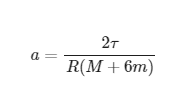where

τ - 200Nm engine torque provided on each wheel
M - 1000 kg mass of the car without wheels
m - 15kg mass of each wheel

After plugging in the numbers

the a=1,048 m/s^2

If a=V/t in the uniform accelerated motion, the t=V/a.
As I'm trying to figure out 0-100km/h acceleration (27,777m/s), after plugging in the numbers

t=26,5sec............ which is very long.

I know that we are discussing a theoretical situation here, but if friction nor such factors as transmission losses are considered here, the more this looks really long. An average car with these parameters (400Nm, 1000kg) would make it in 6-7sec with ease.
I'm wondering if I'm missing something here or if I'm doing something wrong.

I'd be comparing the results with a car on 12kg wheels to see what the difference is but I need to know if I'm doing it right first :D

thanks!

#### Attachments

Homework Helper
Gold Member
You are using engine torque. The formula requires wheel torque. To get wheel torque you need to multiply by the gear ratio for first gear. That will considerably reduce the time required.

•kielbasa, PeroK and Lnewqban
kielbasa
Ok, thanks, that was my initial thought.
To be able to solve this problem, I found this.
nw stands for the number of wheels being driven which I'll discuss in the end.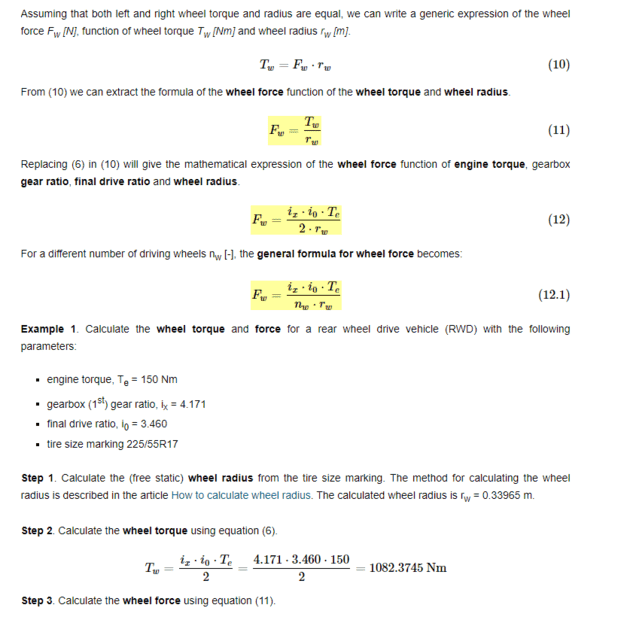If I understand right - total acceleration force does not depend on the number of wheels being driven and it breaks down into the number of wheels, so no matter if we have fwd or 4wd, the total force stays the same (of course such factors as friction, and grip are not considered here). In this case, we are not calculating each torque wheel separately but the total amount of torque transferred to the wheels and converted by the gearbox ratio. Hence we should get rid of the '2' which stands for the number of wheels in the denominator in the above equation

So is my thinking right -
if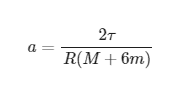from my first post, we should replace τ with the Tw from the previous equation so the final formula should look like this, am I right: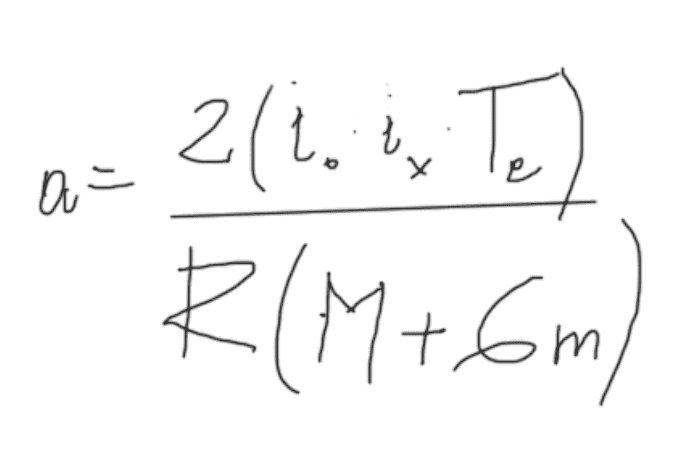?

#### Attachments

kielbasa
Andrewkirk, You said to 'multiply by the first gear ratio'.
Assuming we will be using at least two gears to accelerate from 0-100, shouldn't it be multiplied by the first two gears, not the first one only? I my above formula correct then?

Homework Helper
It is unfortunate that the relevant equations have been presented as bit-mapped screen shots rather than as editable text or typeset ##\LaTeX##. I do not believe that the factor of 2 that is present in your final equation is proper.

Nor do I believe that the idea of a constant torque engine with no maximum RPM limit is particularly realistic. However, let us accept that idea and proceed with a step by step derivation. We are trying to arrive at the formula:$$a = \frac{i_0 \cdot i_x \cdot T_e}{R \cdot (M + 6m)}$$As I understand it, the numerator takes the engine torque multipled by the gearbox ratio to arrive at driveshaft torque. It then takes driveshaft torque times the torque multiplier at the differential to get torque at the wheel(s).

A classic rear wheel differential consists of a bevelled pinion gear mounted on the drive shaft mating at a 90 degree angle with a bevelled ring gear. The ring gear is mounted coaxial with the two axle shafts leading to the wheels. The axle shafts are joined to the ring gear with a torque sharing mechanism so that the sum of the torques at each wheel is equal to the torque the pinion gear applies on the ring gear. For normal straight-line driving, the torque is shared 50/50.
http://www.4x4abc.com/4WD101/img/difff.jpg said: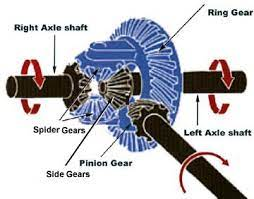This means that the per-wheel torque is halved from ring gear torque. Which means that per-wheel traction force is halved as well. But we are after total force. So we will end up multiplying by the number of driven wheels. The result is a wash. Aside from the pinion gear/ring gear ratio, the presence of the differential has no net effect on the results of our calculation for total force or total acceleration.

We may as well pretend that we have one wheel drive. We have torque at the wheel given by ##i_0 \cdot i_x \cdot T_e##. Force at the wheel is torque divided by the wheel+tire radius ##R##. That accounts for the factor of R. So we have:$$F_\text{tot} = \frac{i_0 \cdot i_x \cdot T_e}{R}$$Effective vehicle mass is given by (for a somewhat reasonable assumption about wheel+tire mass distribution) ##M+4\cdot m \cdot 1.5##. Obviously, this simplifies to ##M+6m##.

The ##4m## is for the total mass of the four wheels. The multiplier of 1.5 is because the wheels are rolling weight. The total kinetic+rotational energy per unit mass goes as ##\frac{3}{4}mv^2## instead of ##\frac{1}{2}mv^2## as long as the mass distribution is like a uniform disc of radius R and we are rolling without slipping. That's the multiplier of 1.5

##F=ma## so ##a = \frac{F}{m}##. We've already decided that the effective mass is ##M+6m## and that the total force is equal to ##\frac{i_0 \cdot i_x \cdot T_e}{R}## so we put it all together and get$$a = \frac{i_0 \cdot i_x \cdot T_e}{R \cdot (M + 6m)}$$
Note that if you measure total vehicle weight ##M## by weighing the vehicle with the wheels on then you've already counted ##4m## as part of ##M##. In that case, the effective vehicle mass is ##M+2m## instead.

Last edited:
•Spinnor and kielbasa
Homework Helper
Andrewkirk, You said to 'multiply by the first gear ratio'.
Assuming we will be using at least two gears to accelerate from 0-100, shouldn't it be multiplied by the first two gears, not the first one only? I my above formula correct then?
You will use the first gear ratio until you reach a speed where the engine reaches red line or a more optimal shift point.

Then you will use the second gear ratio instead. Until the engine reaches red line again or an appropriate shift point into a third gear is reached.

kielbasa
jbriggs444, I appreciate Your help and the explanation. Thank You.
I'm sorry for the bitmaps within the post but it's my first thread and I do not know yet how to properly write down formulas on the forum as You did.
Anyways, the whole equation is copied from another thread I linked up in my first post.
For the first while, I thought '2' in the numerator stood for the two wheels, but that doesn't make sense then.

The whole idea of this thread was to somehow prove, or rather verify, if changing wheels to slightly lighter ones in an average car may have any impact on its acceleration ;) Just my curiosity.
Tried to plug in the numbers real quick and it seems that changing a 15kg wheels to a 12kg ones might result in some slight time improvements. Not by much, but always something.

Mentor
I do not know yet how to properly write down formulas on the forum as You did.
Please see the "LaTeX Guide" link below the Edit window for a nice introduction.if changing wheels to slightly lighter ones in an average car may have any impact on its acceleration
Yes, lighter is better for angular acceleration and also for unsprung weight (for handling).

changing a 15kg wheels to a 12kg ones might result in some slight time improvements
Yes, it should for acceleration, depending on other factors like wheel diameter, etc.

EDIT/ADD -- but keep in mind that wheel mass and Moment of Inertia are a much smaller influence on the acceleration of a car versus a motorcycle or bicycle. You just need to do the calculations to see what the magnitude of improvement is, based on the overall mass of your vehicle.

Last edited:
•Lnewqban, kielbasa and jbriggs444
Homework Helper
•berkeman
kielbasa
Thanks..looks clear then.

•berkeman
Homework Helper
If the wheel and tire approximate a hoop (like a bicycle tire), the affect on acceleration would be equivalent to a non-rotating object with 2.0 times the mass. If the wheel and tire approximate a solid disk, the affect on acceleration would be equivalent to a non-rotating object with 1.5 times the mass.

•jbriggs444
zanick
Hi,

https://www.physicsforums.com/threa...g-the-moment-of-inertia-of-the-wheels.930374/
but as it has been closed to replies, I decided to ask here.

The thread ended up with the equation:

View attachment 322867

where

τ - 200Nm engine torque provided on each wheel
M - 1000 kg mass of the car without wheels
m - 15kg mass of each wheel

After plugging in the numbers

the a=1,048 m/s^2

If a=V/t in the uniform accelerated motion, the t=V/a.
As I'm trying to figure out 0-100km/h acceleration (27,777m/s), after plugging in the numbers

t=26,5sec............ which is very long.

I know that we are discussing a theoretical situation here, but if friction nor such factors as transmission losses are considered here, the more this looks really long. An average car with these parameters (400Nm, 1000kg) would make it in 6-7sec with ease.
I'm wondering if I'm missing something here or if I'm doing something wrong.

I'd be comparing the results with a car on 12kg wheels to see what the difference is but I need to know if I'm doing it right first :D

thanks!
Having gone over all possible ways to make my race car perform better, this has come up a number of times with racers, and i have answered them with a much easier description than outlined here . if you think about it, there is a way to equate the rotational mass to what it would equate to if it was sitting in the car, then just take your HP/weight ratios to figure out the effects. generally, on a 17" wheel with mass evenly distributed, the weight of the wheel and tire, has an effect as if 1.4 x the weight was in the car passenger area (. 20" wheels and tires, it might be 2x.) The next thing to consider would be the unsprung weight effect, which is a handling issue. In the end , its clear to see that the HP gained by lighter wheels (overall acceleration capacity at any vehicle speed) is pretty small. say you have a 60lb wheel and tire, and lost 10lbs per corner.... that would be 40lbs (x.1.5) =60lbs effective translational mass savings..... that might be a 6hp gain. (300rwhp , 3000lb weight car ) HP is the best way to quickly understand the effects , because by definition it actually gives the value of the cars ability to accelerate at any speed. rate of change of kinetic energy. it's how dynos measure cars HP as well.

Homework Helper
on a 17" wheel with mass evenly distributed, the weight of the wheel and tire, has an effect as if 1.4 x the weight was in the car passenger area (. 20" wheels and tires, it might be 2x.)
The multiplier is independent of tire radius. Only the tire's mass distribution enters in. An even weight distribution gives a 1.5 to one multiplier regardless.

Double the tire radius and you double the moment of inertia. But you also halve the rotation rate. Since rotational kinetic energy goes as ##\frac{1}{2}I\omega^2##, the result is a wash.

You cannot exceed a multiplier of 2 to 1 without adding spikes (or similar appendages) that extend beyond the nominal radius.

zanick
The multiplier is independent of tire radius. Only the tire's mass distribution enters in. An even weight distribution gives a 1.5 to one multiplier regardless.

Double the tire radius and you double the moment of inertia. But you also halve the rotation rate. Since rotational kinetic energy goes as ##\frac{1}{2}I\omega^2##, the result is a wash.

You cannot exceed a multiplier of 2 to 1 without adding spikes (or similar appendages) that extend beyond the nominal radius.
I was rounding but yes, 1/2Iw^2..... but also 1/2M(wxR)^2 So we then can solve for M which is M=I/R^2 . So it will vary for wheel sizes 13" to 18" for example for values ranging from 1.3 to near 1.5. and going to 2x (as you indicated) when just talking about adding weight to the outer "donut" or tire.

It gets a little more complicated when taking this weight of a flywheel before the gear box, then the implications are far greater in the lower gears . for moderate weight savings on the flywheel, it could be like removing 200lbs from the car in 1st gear while only being like 10lbs removed from the car in 5th gear both for a 7lbs shaving of weight off the flywheel.

•jbriggs444
But ##\omega## is a function of ##R##:$$\omega = \frac{v}{R}$$If you write things in terms of ##v## this means that your ##\frac{1}{2}I\omega^2## becomes $$\frac{1}{4}Mv^2$$for a disc with a uniform mass distribution. This is independent of ##R##. You can then see where the 1.5 multiplier comes from:$$E_\text{tot} = \frac{1}{2}Mv^2 + \frac{1}{4}Mv^2 = 1.5 ( \frac{1}{2}Mv^2 )$$